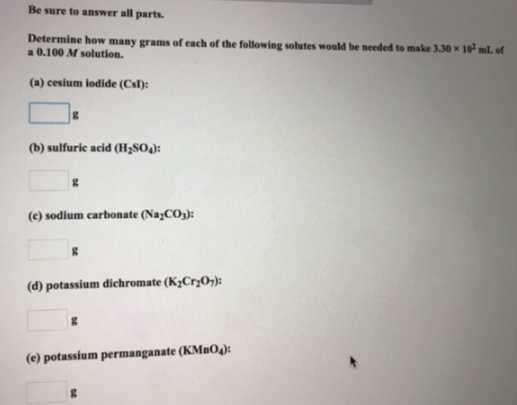# Problem: Be sure to answer all parts. Determine how many grams of each of the following solutes would be needed to make 3.30 x 102 mL of a 0.100 M solution. (a) cesium iodide (Csl): (b) sulfuric acid (H2SO4): (c) sodium carbonate (Na2CO3): (d) potassium dichromate (K2Cr2O): (e) potassium permanganate (KMnO4):

###### FREE Expert Solution
89% (130 ratings)###### Problem Details

Be sure to answer all parts.

Determine how many grams of each of the following solutes would be needed to make 3.30 x 102 mL of a 0.100 M solution.

(a) cesium iodide (Csl):

(b) sulfuric acid (H2SO4):

(c) sodium carbonate (Na2CO3):

(d) potassium dichromate (K2Cr2O):

(e) potassium permanganate (KMnO4):